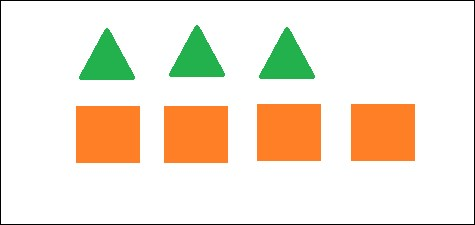•#### Big Data Analytics with Java#### Overview of this book

This book covers case studies such as sentiment analysis on a tweet dataset, recommendations on a movielens dataset, customer segmentation on an ecommerce dataset, and graph analysis on actual flights dataset. This book is an end-to-end guide to implement analytics on big data with Java. Java is the de facto language for major big data environments, including Hadoop. This book will teach you how to perform analytics on big data with production-friendly Java. This book basically divided into two sections. The first part is an introduction that will help the readers get acquainted with big data environments, whereas the second part will contain a hardcore discussion on all the concepts in analytics on big data. It will take you from data analysis and data visualization to the core concepts and advantages of machine learning, real-life usage of regression and classification using Naïve Bayes, a deep discussion on the concepts of clustering,and a review of simple neural networks on big data using deepLearning4j or plain Java Spark code. This book is a must-have book for Java developers who want to start learning big data analytics and want to use it in the real world.
Big Data Analytics with JavaCreditswww.PacktPub.comCustomer FeedbackPrefaceFree Chapter
Big Data Analytics with JavaFirst Steps in Data AnalysisData VisualizationBasics of Machine LearningRegression on Big DataNaive Bayes and Sentiment AnalysisDecision TreesEnsembling on Big DataRecommendation SystemsClustering and Customer Segmentation on Big DataMassive Graphs on Big DataReal-Time Analytics on Big DataDeep Learning Using Big DataIndex## Conditional probability

Conditional probability in simple terms is the probability of occurrence of an event given that another event has already occurred. It is given by the following formula:

P(B|A)= P(A and B)/P(A)

Here in this formula the values stand for:

Probability value

Description

P(B|A)

This is the probability of occurrence of event B given that event A has already occurred.

P(A and B)

The probability that both event A and B occur.

P(A)

This is the probability of occurrence of an event A.

Now let's try to understand this using an example. Suppose we have a set of seven figures as follows:As seen in the preceding figure, we have three triangles and four rectangles. So if we randomly pull one figure from this set the probability that it belongs to either of the figures will be:

P(triangle) = Number of Triangles / Total number of figures = 3 / 7

P(rectangle) = Number of rectangles / Total number of figures = 4 / 7

Now suppose we break the figure into two individual sets...